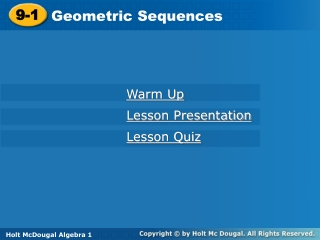Download Presentation9-1

# 9-1

Download Presentation## 9-1

- - - - - - - - - - - - - - - - - - - - - - - - - - - E N D - - - - - - - - - - - - - - - - - - - - - - - - - - -
##### Presentation Transcript

1. 9-1 Geometric Sequences Warm Up Lesson Presentation Lesson Quiz Holt McDougal Algebra 1 Holt Algebra 1

2. Objectives Recognize and extend geometric sequences. Find the nth term of a geometric sequence.

3. 4 4 4 Example 1A Continued Find the next three terms in the geometric sequence. 1, 4, 16, 64,… Step 2 Multiply each term by 4 to find the next three terms. 64 256 1024 4096 The next three terms are 256, 1024, and 4096.

4. Step 2 Multiply each term by to find the next three terms. The next three terms are Example 1B Continued Find the next three terms in the geometric sequence.

5. Check It Out! Example 1b Continued Find the next three terms in the geometric sequence. 512, 384, 288,… Step 2 Multiply each term by 0.75 to find the next three terms. 288 216 162 121.5 0.75 0.75 0.75 The next three terms are 216, 162, and 121.5.

6. nth term 1st term Common ratio If the first term of a geometric sequence is a1, the nth term is an , and the common ratio is r, then an=a1rn–1

7. Example 2A: Finding the nth Term of a Geometric Sequence The first term of a geometric sequence is 500, and the common ratio is 0.2. What is the 7th term of the sequence? an = a1rn–1 Write the formula. a7 = 500(0.2)7–1 Substitute 500 for a1,7 for n, and 0.2 for r. Simplify the exponent. = 500(0.2)6 Use a calculator. = 0.032 The 7th term of the sequence is 0.032.

8. Example 2B: Finding the nth Term of a Geometric Sequence For a geometric sequence, a1 = 5, and r = 2. Find the 6th term of the sequence. an = a1rn–1 Write the formula. a6 = 5(2)6–1 Substitute 5 for a1,6 for n, and 2 for r. = 5(2)5 Simplify the exponent. = 160 The 6th term of the sequence is 160.

9. 2 –6 18 –54 Example 2C: Finding the nth Term of a Geometric Sequence What is the 9th term of the geometric sequence 2, –6, 18, –54, …? The value of r is –3. an = a1rn–1 Write the formula. Substitute 2 for a1,9 for n, and –3 for r. a9 = 2(–3)9–1 = 2(–3)8 Simplify the exponent. Use a calculator. = 13,122 The 9th term of the sequence is 13,122.

10. 300 150 75 Example 3: Application A ball is dropped from a tower. The table shows the heights of the balls bounces, which form a geometric sequence. What is the height of the 6th bounce? The value of r is 0.5.

11. Example 3 Continued an = a1rn–1 Write the formula. Substitute 300 for a1, 6 for n, and 0.5 for r. a6 = 300(0.5)6–1 = 300(0.5)5 Simplify the exponent. Use a calculator. = 9.375 The height of the 6th bounce is 9.375 cm.

12. Check It Out! Example 3 The table shows a car’s value for 3 years after it is purchased. The values form a geometric sequence. How much will the car be worth in the 10th year? 10,000 8,000 6,400 The value of r is 0.8.

13. Check It Out! Example 3 an = a1rn–1 Write the formula. Substitute 10,000 for a1,10 for n, and 0.8 for r. a6 = 10,000(0.8)10–1 = 10,000(0.8)9 Simplify the exponent. Use a calculator. = 1,342.18 In the 10th year, the car will be worth \$1342.18.

14. Lesson Quiz: Part I Find the next three terms in each geometric sequence. 1. 3, 15, 75, 375,… 2. 3. The first term of a geometric sequence is 300 and the common ratio is 0.6. What is the 7th term of the sequence? 4. What is the 15th term of the sequence 4, –8, 16, –32, 64…? 1875; 9375; 46,875 13.9968 65,536

15. Lesson Quiz: Part II Find the next three terms in each geometric sequence. 5. The table shows a car’s value for three years after it is purchased. The values form a geometric sequence. How much will the car be worth after 8 years? \$5570.39